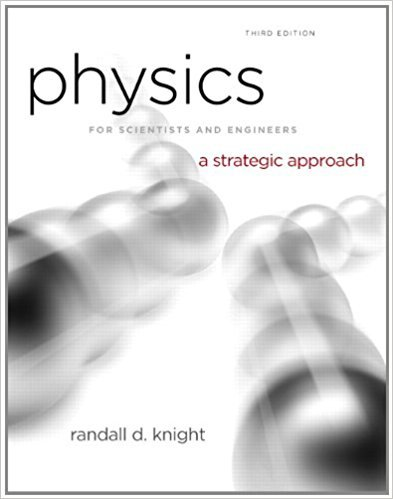×
Log in to StudySoup
Get Full Access to Physics For Scientists And Engineers: A Strategic Approach With Modern Physics - 3 Edition - Chapter 1 - Problem 49p
Join StudySoup for FREE
Get Full Access to Physics For Scientists And Engineers: A Strategic Approach With Modern Physics - 3 Edition - Chapter 1 - Problem 49p

Already have an account? Login here
×
Reset your password

# show a partial motion diagram. For:a. Complete the motionISBN: 9780321740908 69

## Solution for problem 49P Chapter 1

Physics for Scientists and Engineers: A Strategic Approach with Modern Physics | 3rd Edition

• Textbook Solutions
• 2901 Step-by-step solutions solved by professors and subject experts
• Get 24/7 help from StudySoup virtual teaching assistantsPhysics for Scientists and Engineers: A Strategic Approach with Modern Physics | 3rd Edition

4 5 1 427 Reviews
15
4
Problem 49P

Problem 49P

Problem show a partial motion diagram. For:

a. Complete the motion diagram by adding acceleration vectors.

b. Write a physics problem for which this is the correct motion diagram. Be imaginative! Don’t forget to include enough information to make the problem complete and to state clearly what is to be found.

c. Draw a pictorial representation for your problem.

FIGUREStep-by-Step Solution:

Solution 49 P:

Step 1 of 2:-

a)

Here we have to complete the motion diagram given, by adding the acceleration vectors.Here the velocity is decreasing with time, so the acceleration is in the opposite direction of motion.

b)

Here we have to write a story which resembles with the motion diagram.

This can be considered as, a picture of a ball thrown upwards with some initial velocity, which stops at a maximum height.

Here we are not showing, what happens after once the ball reached the maximum height.

The picture and the story ends as the ball makes it to the highest point. The acceleration is the acceleration due to gravity.

Step 2 of 2

##### ISBN: 9780321740908

Unlock Textbook Solution

Enter your email below to unlock your verified solution to:

show a partial motion diagram. For:a. Complete the motion